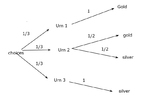# Probability (Bayes' formula)

• stunner5000pt

#### stunner5000pt

Homework Statement
There are three urn contains coins
Urn 1: 5 gold coins
Urn 2: 3 gold, 3 silver
Urn 3: 3 silver

An urn is selected at randonm & a coin is drawn from the urn. If the selected coin is silver, what is the probability that urn III was selected

Determine the probability using Bayes formula & a tree
Relevant Equations
Bayes Formula
From my understanding of Bayes formula, it should look like something like this

$$P(Silver| III) = \frac{P(III | silver) \times P(silver)}{P(III)}$$

now we know that P(urn III) = 1/3
and the probability of P(silver) = Pr(silver|urn I) + P(silver|urn II) + P(silver|urn III) = 1/3 (0) + 1/3 (1/2) + 1/3 (1) = 1/2

But how do i calculate P(urn 3|silver) ? Would it simply be 1/3?

If I used this, then
P(Silver| III) = (1/3)(1/2) / (1/3) = 1/2. is this correct?

Using the tree, I have attached what I believe is the right tree.
Would the answer then be P(silver| urn III) = 1/3 / (1/2) = 2/3 ?

#### Attachments

•Capture.PNG
8.4 KB · Views: 46
It's not clear to me whether you are trying to calculate the probability of urn 3 given silver;or, silver given urn 3.

The probability tree is the way to go, IMO. But you need to combine probabilities as you go along. The last column in the tree should sum to 1, not 2 ( as you have).

PeroK said:
It's not clear to me whether you are trying to calculate the probability of urn 3 given silver;or, silver given urn 3.

The probability tree is the way to go, IMO. But you need to combine probabilities as you go along. The last column in the tree should sum to 1, not 2 ( as you have).
I believe that my usage of the Bayes formula (A|B) might be throwing you off.

The question statement is " An urn is selected at random & a coin is drawn from the urn. If the selected coin is silver, what is the probability that urn III was selected"

if using the formula, would it be the 1/2 that I got?

In the tree, doesn't it all add to 1? That is the
top probability is for urn 1 is 1/3, \
the middle two for urn 2 are 1/6 each and if you add them together you get 1/3,
and the bottom is 1/3

so if we add the 3(1/3) = 1?

stunner5000pt said:
I believe that my usage of the Bayes formula (A|B) might be throwing you off.

The question statement is " An urn is selected at random & a coin is drawn from the urn. If the selected coin is silver, what is the probability that urn III was selected"

if using the formula, would it be the 1/2 that I got?
I don't think so.
stunner5000pt said:
In the tree, doesn't it all add to 1?
it doesn't. The last column adds up to 2.
stunner5000pt said:
That is the
top probability is for urn 1 is 1/3, \
the middle two for urn 2 are 1/6 each and if you add them together you get 1/3,
and the bottom is 1/3

so if we add the 3(1/3) = 1?
Those numbers are missing from your tree.

In any case, how do you interpret your tree?

•stunner5000pt
stunner5000pt said:
Homework Statement:: There are three urn contains coins
Urn 1: 5 gold coins
Urn 2: 3 gold, 3 silver
Urn 3: 3 silver

An urn is selected at randonm & a coin is drawn from the urn. If the selected coin is silver, what is the probability that urn III was selected

Determine the probability using Bayes formula & a tree
Relevant Equations:: Bayes Formula

From my understanding of Bayes formula, it should look like something like this

$$P(Silver| III) = \frac{P(III | silver) \times P(silver)}{P(III)}$$

now we know that P(urn III) = 1/3
Yes.
stunner5000pt said:
and the probability of P(silver) = Pr(silver|urn I) + P(silver|urn II) + P(silver|urn III) = 1/3 (0) + 1/3 (1/2) + 1/3 (1) = 1/2
You mean P(silver) = P(silver|urn I)P(urn I) + P(silver|urn II)P(urn II) + P(silver|urn III)P(urn III), which is what you calculated.
EDIT to match his calculations: P(silver) = P(urn I)P(silver|urn I) + P(urn II)P(silver|urn II) + P(urn III)P(silver|urn III)
stunner5000pt said:
But how do i calculate P(urn 3|silver) ? Would it simply be 1/3?
It is whatever you get when you plug the numbers into the Relevant Equation you gave us.
Rearrange the Relevant Equation to get P(urn III | silver) = P(silver | urn III)P(urn III) / P(silver).
stunner5000pt said:
If I used this, then
P(Silver| III) = (1/3)(1/2) / (1/3) = 1/2. is this correct?
No. P(urn III | silver) = P(silver | urn III)P(urn III) / P(silver) = ???.
stunner5000pt said:
Using the tree, I have attached what I believe is the right tree.
Would the answer then be P(silver| urn III) = 1/3 / (1/2) = 2/3 ?
Yes,
CORRECTION: I think you mean P(urn III | silver = 2/3. That is what the problem asked for.

Last edited:
•stunner5000pt
stunner5000pt said:
Using the tree, I have attached what I believe is the right tree.
Would the answer then be P(silver| urn III) = 1/3 / (1/2) = 2/3 ?
FactChecker said:
Yes.
Except ##P(silver| III) =1##, as that is the probability of silver given urn III.

•FactChecker and stunner5000pt
PeroK said:
Except ##P(silver| III) =1##, as that is the probability of silver given urn III.
Thanks! I stand corrected. I'm sure that he meant P( urn III | silver), which is what the problem asked for. I corrected my post.

•stunner5000pt